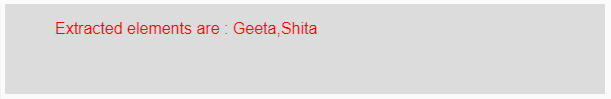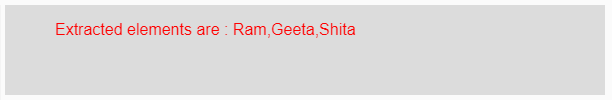# p5.js | subset() function

The subset() function in p5.js is used to get the subset of the given array elements. This function extracts the elements from an existing array.

Syntax:

`subset(Array, Start, Count)`

Parameters: This function accepts three parameters as mentioned above and described below:

• Array: This parameter holds the array elements on which the subset() operation is to be done to get its subset element.
• Start: This is the number from where extraction is to be done. It’s value starts from 0.
• Count: This is the number of elements which are to be extracted.

Return Value: It returns the extracted elements.

Below programs illustrate the subset() function in p5.js:

Example 1: This example uses subset() function to get the subset of the given array elements.

 `function` `setup() {  ` ` `  `    ``// Creating Canvas size ` `    ``createCanvas(600, 90);  ` `}  ` ` `  `function` `draw() {  ` `     `  `    ``// Set the background color  ` `    ``background(220);  ` `     `  `    ``// Initializing the array ` `    ``let Array = [``'Ram'``, ``'Geeta'``, ``'Shita'``, ``'Shyam'``]; ` `   `  `    ``// Initializing a start value form where ` `    ``// extraction is done ` `    ``// it starts form 0. ` `    ``let Start = 1; ` `   `  `    ``// Initializing the count which defines ` `    ``// the number of elements to be extracted  ` `    ``let Count = 2; ` `   `  `    ``// Calling to subset() function. ` `    ``let A = subset(Array, Start, Count); ` `     `  `    ``// Set the size of text  ` `    ``textSize(16);  ` `     `  `    ``// Set the text color  ` `    ``fill(color(``'red'``));  ` `   `  `    ``// Getting extracted elements ` `    ``text(``"Extracted elements are : "` `+ A, 50, 30);           ` `}  `

Output:Example 2: This example uses subset() function to get the subset of the given array elements.

 `function` `setup() {  ` ` `  `    ``// Creating Canvas size ` `    ``createCanvas(600, 90);  ` `}  ` ` `  `function` `draw() {  ` `     `  `    ``// Set the background color  ` `    ``background(220);  ` `     `  `    ``// Initializing the array ` `    ``let Array = [``'Ram'``, ``'Geeta'``, ``'Shita'``, ``'Shyam'``]; ` `   `  `    ``// Initializing a start value form where ` `    ``// extraction is done ` `    ``// it starts form 0. ` `    ``let Start = 0; ` `   `  `    ``// Initializing the count which defines ` `    ``// the number of elements to be extracted  ` `    ``let Count = 3; ` `   `  `    ``// Calling to subset() function. ` `    ``let A = subset(Array, Start, Count); ` `     `  `    ``// Set the size of text  ` `    ``textSize(16);  ` `     `  `    ``// Set the text color  ` `    ``fill(color(``'red'``));  ` `   `  `    ``// Getting extracted elements ` `    ``text(``"Extracted elements are : "` `+ A, 50, 30);            ` `}  `

Output:Reference: https://p5js.org/reference/#/p5/subset

My Personal Notes arrow_drop_upCheck out this Author's contributed articles.

If you like GeeksforGeeks and would like to contribute, you can also write an article using contribute.geeksforgeeks.org or mail your article to contribute@geeksforgeeks.org. See your article appearing on the GeeksforGeeks main page and help other Geeks.

Please Improve this article if you find anything incorrect by clicking on the "Improve Article" button below.

Article Tags :

Be the First to upvote.

Please write to us at contribute@geeksforgeeks.org to report any issue with the above content.